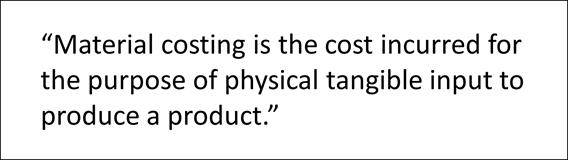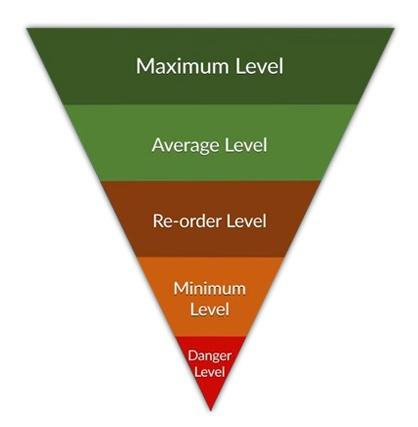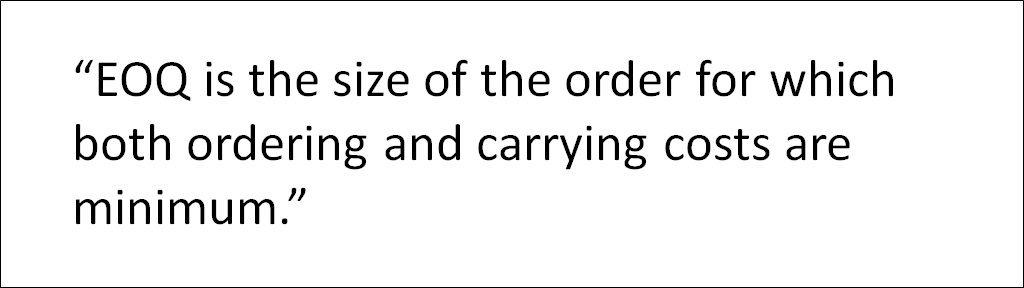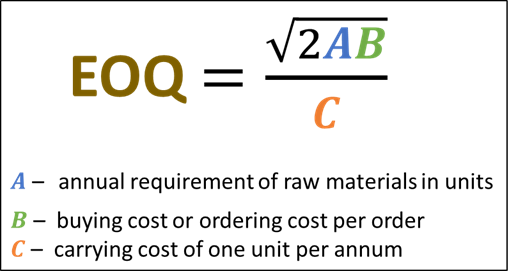# What is Material Costing?you may recall that costing is nothing but the process of ascertaining or determining the expenditure incurred for producing a product or rendering a service. From the manufacturer’s point of view, of course. (If you want a brief overview of concepts in costing, look at this post.)

And as part of this determination, you may want to identify what are the costs of materials to produce a product. This process is known as material costing. Let us explore some of the concepts under material costing in depth.

### What is Material Costing?

The formal definition of material costing is as follows:The definition is a bit wordy, so let us break this down.

The first part of the definition is clear enough: cost incurred for the purpose of …

It gets confusing when we get to physical tangible input. What in the world is physical tangible input?

It is a simple concept.

You can think of physical tangible input as the input to produce a product. The input is the raw material used in the product. This is where the term material costing comes from.

Clear enough.

But what are the strategies or techniques involved in material costing?

To understand that, we need to know what the main objectives are in material costing. Let us go through them briefly.

Objectives of Material Costing

Before going through the objectives of material costing, you may want to recall the objectives of costing itself.

These are:

• Ascertainment of cost
• Determination of selling price
• Ascertainment of profit on each activity
• Cost control
• Cost reduction
• Decision making

In material costing, the main objectives are cost control and cost reduction.

In this post, we will look at a subset of these objectives. Cost minimisation.

In other words, how are we going to minimise the cost related to material cost?

There are many ways to reduce such cost. One technique is known as Inventory Control.

Inventory Control

This is a vast topic in and in of itself. The idea is to manage or control your inventory. And of course, there are various techniques under inventory control. Collectively, these are called inventory control techniques.

The techniques are:

• Purchase procedures
• Material accounting procedures
• Setting of Various Stock Levels
• Economic Ordering Quantity (EOQ)
• ABC Analysis
• Review of slow moving and non-moving stock
• Two Bin system
• Perpetual Inventory records & Continuous Stock Verification
• Physical Stock Verification
• Budgetary control
• Ratio analysis

In this post, we are not going through all the techniques in detail. No. That is beyond the scope of this brief survey.

Instead, we will give you an overview of three of these methods. That way, you will understand that the concepts under costing are not all that difficult. And that to do well in the costing paper, especially in regard to material costing, you need to have common sense. As well as a thorough understanding of concepts.

The three methods we will look at are setting of various stock levels, economic ordering quantity (EOQ) and ABC analysis.

Setting of Various Stock Levels

The idea behind this technique is to identify what levels your stocks are so that you can minimise the costs involved in inventory. The diagram below shows the stock levels.As you can see there are five stock levels. To identify which level your stock is, you need to make use of certain formulae.

The table below shows this:

 Stock Level Method of Calculation Maximum Level Re-order level + Re-order quantity – (Minimum Usage * Minimum Lead time) Average Level (Maximum level + minimum level)/2                           OR Minimum level + ½(Re-order quantity) Re-order Level Maximum Usage * Maximum Lead Time Minimum Level Re-order level – (Average usage * average lead time) Danger Level Minimum Usage * Minimum Lead Time           OR Minimum Usage * Minimum Time for Emergency Purchase

Notice that you cannot calculate the stock levels independently of each other. For example, to calculate what your maximum level is, you will have to first calculate your re-order level.

Also, at this point, you may not know what the terms minimum lead time, maximum usage, etc. are. But you probably could guess, I think! In any case, as we said, this post is meant to be a brief survey of the techniques. If you do want to know more about these terms, why don’t you sign up forThat way, you will then get an in depth understanding of these concepts.

Right.

Sorry for the digression.

The main takeaway here is that to identify the levels in stock, you will need to apply certain formulae. And these formulae need to be understood and memorised to do well in the exam.

Now, let us go through the next topic in our discussion. The concept of Economic Ordering Quantity or EOQ.

Economic Ordering Quantity (EOQ)

As always, let us begin with a definition.Note the terms ordering costs and carrying costs.

Ordering costs are also known as buying costs. These are costs associated with creating and processing orders to suppliers.

Carrying costs, also called stock holding costs, are the costs of holding items in inventory or stock.

And as always, we are not going to go in detail the meaning of these terms. It is quite self-explanatory.

There are two ways to compute EOQ. They are:

• Formula-based method
• Concept-based method (Tabular Method)

We use either of these methods depending on the situation. We use the formulae-based method when there is no discount or no change in the purchase price. We use the tabular method if the purchase price changes or there is a discount involved.

We will go over the formula-based method here.

EOQ: Formula-based method

R.H. Wilson was a consultant who developed and used this method of determining EOQ.

Before going into the determination of EOQ, let us look at what exactly is going on.

Remember that in this topic of material costing,

 Total cost Purchase value of raw material + associated cost Associated cost Ordering cost + carrying cost

We want to find out the minimum ordering cost and carrying cost. The order quantity that does this, as we said before, is EOQ.

It is given by (assuming no discount or no change in purchase price):For the exam, you will need to know how to use this formula and its applicability.

So, that is EOQ in a nutshell. Let us now go over one last topic under inventory control. ABC Analysis.

ABC Analysis

ABC Analysis has several alternate names: Pareto analysis, selective stock control analysis or 70 – 20 – 10 analysis.

It is quite simple really.

It states that all the companies’ stocks should be classified into three categories, A, B and C.

The stock under category A contains items that are of high value (price) but low quantity (number of items).

The stock under category C contains items of low value but high quantity.

The stock under category B contains items whose value and quantity lie “somewhere in between” the two extremes.

These terms high quantity, low quantity, high value, low value and even “somewhere in between” have specific meanings.

In this context, high value means those items whose cost makes up 70% of the total cost. Low quantity means that the number of these items in stock make up only 10% of the total.

Likewise, high quantity means that the number of these items in stock make up 70% of the total. And low value means that the cost is 10% of the total.

And the “somewhere in between” values are items whose cost is 20% of the total and quantity 20% of the total.

The table below outlines all this.

 ABC Analysis A B C Value 70% 20% 10% Quantity 10% 20% 70%

As you can see, that is why this form of analysis is also known as 70-20-10 analysis!

Classifying and categorising stock in this way gives a better control over the stock.

Summary

Okay, let us now summarise this post. We talked about the definition of material costing and saw that it is the process of identifying the costs of material needed to produce a product. We then looked at the formal definition. Following this, we found that one of the techniques involved in material costing is inventory control. Under inventory control, we looked at three methods: setting of various stock levels, EOQ and ABC analysis. Although we did not go over these methods in depth, we did get a general idea of the techniques involved.

Want to know more about what material costing entails? Check out this video by CA Hariharan where he gives you an overview of the concepts we talked about in this post. Also, if you want an in-depth explanation of material costing, why don’t you sign up forThat way, you will be ready to conquer the exam with no fear.